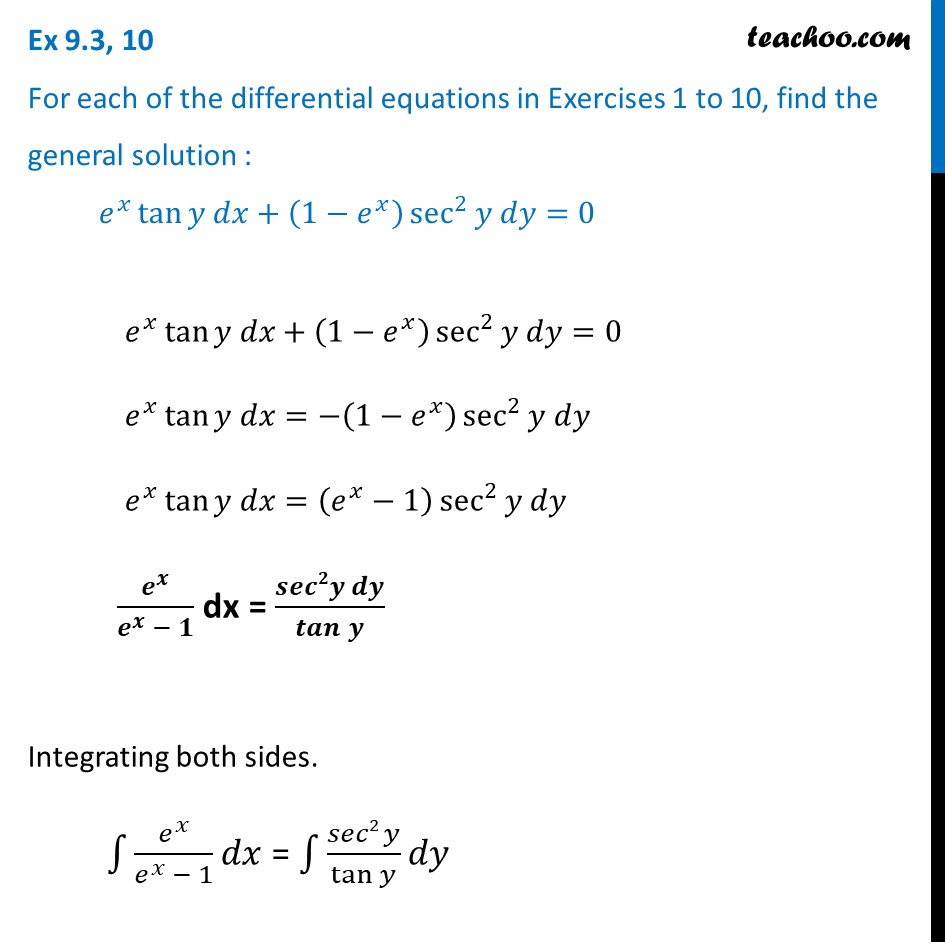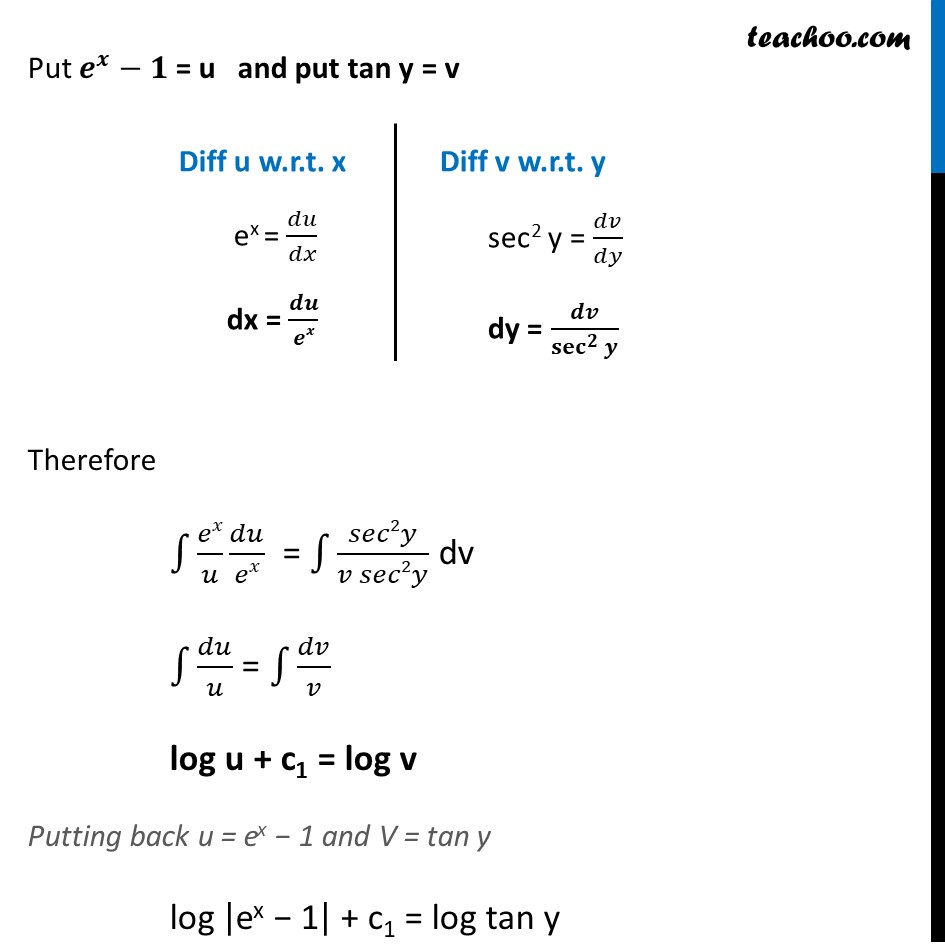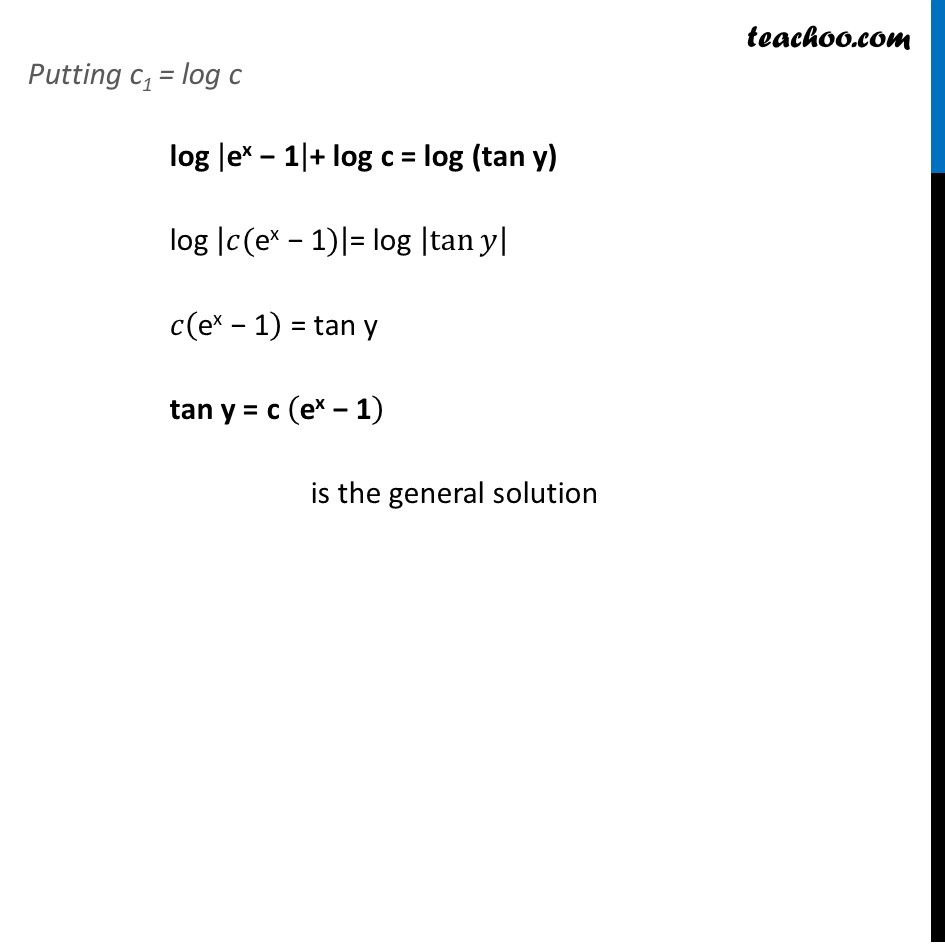Ex 9.3

Chapter 9 Class 12 Differential Equations
Serial order wiseLearn in your speed, with individual attention - Teachoo Maths 1-on-1 Class

### Transcript

Ex 9.3, 10 For each of the differential equations in Exercises 1 to 10, find the general solution : 𝑒^𝑥 tan⁡〖𝑦 𝑑𝑥+(1−𝑒^𝑥 ) sec^2⁡〖𝑦 𝑑𝑦=0〗 〗 𝑒^𝑥 tan⁡〖𝑦 𝑑𝑥+(1−𝑒^𝑥 ) sec^2⁡〖𝑦 𝑑𝑦=0〗 〗 𝑒^𝑥 tan⁡〖𝑦 𝑑𝑥=−(1−𝑒^𝑥 ) sec^2⁡〖𝑦 𝑑𝑦〗 〗 𝑒^𝑥 tan⁡〖𝑦 𝑑𝑥〗=(𝑒^𝑥−1) sec^2⁡〖𝑦 𝑑𝑦〗 𝒆^𝒙/(𝒆^𝒙 − 𝟏) dx = (𝒔𝒆𝒄𝟐𝒚 𝒅𝒚)/𝒕𝒂𝒏⁡𝒚 Integrating both sides. ∫1▒〖𝑒^𝑥/(𝑒^𝑥 − 1) 𝑑𝑥〗 = ∫1▒〖(𝑠𝑒𝑐2 𝑦)/tan⁡𝑦 𝑑𝑦〗 Put 𝒆^𝒙−𝟏 = u and put tan y = v Diff u w.r.t. x ex = 𝑑𝑢/𝑑𝑥 dx = 𝒅𝒖/𝒆𝒙 Diff v w.r.t. y sec2 y = 𝑑𝑣/𝑑𝑦 dy = 𝒅𝒗/〖𝐬𝐞𝐜〗^𝟐⁡𝒚 Therefore ∫1▒〖𝑒𝑥/𝑢 𝑑𝑢/𝑒𝑥〗 = ∫1▒𝑠𝑒𝑐2𝑦/(𝑣 𝑠𝑒𝑐2𝑦) dv ∫1▒𝑑𝑢/𝑢 = ∫1▒𝑑𝑣/𝑣 log u + c1 = log v Putting back u = ex − 1 and V = tan y log |"ex − 1" | + c1 = log tan y Putting c1 = log c log |"ex − 1" |+ log c = log (tan y) log |𝑐("ex − 1" )|= log |tan⁡𝑦 | 𝑐("ex − 1" ) = tan y tan y = c ("ex − 1" ) is the general solution